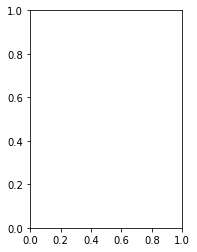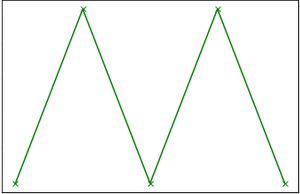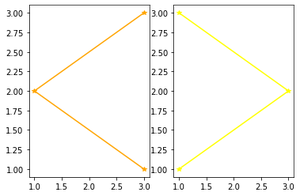Related Articles

# Matplotlib.pyplot.subplot() function in Python

• Last Updated : 11 Dec, 2020

Prerequisites: matplotlib

subplot() function adds subplot to a current figure at the specified grid position.  It is similar to the subplots() function however unlike subplots() it adds one subplot at a time. So to create multiple plots you will need several lines of code with the subplot() function. Another drawback of the subplot function is that it deletes the preexisting plot on your figure. Refer to example 1.

It is a wrapper of Figure.add_subplot.

Syntax:

subplot(nrows, ncols, index, **kwargs)

subplot(pos, **kwargs)

subplot(ax)

Parameters :

• args:  Either a 3-digit integer or three separate integers describing the position of the subplot.
• pos is a three-digit integer where the first, second, and third integer are nrows,ncols, index.
• projection : [{None, ’aitoﬀ’, ’hammer’, ’lambert’, ’mollweide’, ’polar’, ’rectilinear’, str}, optional]. The projection-type of the subplot (Axes). The default None results in a ’rectilinear’ projection.
• label : [str] A label for the returned axes.
• **kwargs: This method also takes the keyword arguments for the returned axes base class;
except for the ﬁgure argument, for e.g facecolor.

Returns :  An axes.SubplotBase subclass of Axes or a subclass of Axes. The returned axes base class depends on the projection used.

Implementation of the function is given below:

Example 1: subplot() will delete the pre-existing plot.

## Python3

 `# importing hte module``import` `matplotlib.pyplot as plt`` ` `# Data to display on plot``x ``=` `[``1``, ``2``, ``3``, ``4``, ``5``]``y ``=` `[``1``, ``2``, ``1``, ``2``, ``1``]`` ` `# plot() will create new figure and will add axes object (plot) of above data``plt.plot(x, y, marker``=``"x"``, color``=``"green"``)`` ` `# subplot() will add plot to current figure deleting existing plot``plt.subplot(``121``)`

Output: We can see that the first plot got set aside by the subplot() function.subplot_gfg

If you want to see the first plot comment out plt.subplot() line and you will see the following plotplot_gfg

Example 2:

## Python3

 `import` `matplotlib.pyplot as plt``# data to display on plots`` ` `x ``=` `[``3``, ``1``, ``3``]``y ``=` `[``3``, ``2``, ``1``]``z ``=` `[``1``, ``3``, ``1``]`` ` `# Creating figure object``plt.figure()`` ` `# addind first subplot``plt.subplot(``121``)``plt.plot(x, y, color``=``"orange"``, marker``=``"*"``)`` ` `# addding second subplot``plt.subplot(``122``)``plt.plot(z, y, color``=``"yellow"``, marker``=``"*"``)`

Output :multiple_subplots

Attention geek! Strengthen your foundations with the Python Programming Foundation Course and learn the basics.

To begin with, your interview preparations Enhance your Data Structures concepts with the Python DS Course. And to begin with your Machine Learning Journey, join the Machine Learning – Basic Level Course

My Personal Notes arrow_drop_up